Math resources Geometry Volume

Volume of a prism

# Volume of a prism

Here you will learn about the volume of a prism, including what it is and how to find it.

Students will first learn about the volume of a prism as part of geometry in 5 th grade. Students will expand on this knowledge in 6 th grade and 7 th grade geometry.

## What is the volume of a prism?

The volume of a prism is the amount of space there is within it.

\text {Volume of prism }=\text { Area of the base } \times \text { height}

For example,

Imagine filling this L-shaped prism fully with water. The total amount of water inside the prism would represent the volume of the prism in cubic units.

To calculate the volume of any prism, we find the area of the base and multiply it by the height.

To identify the base of the prism, look for two opposite congruent sides that are connected by any number of lateral sides. For right prisms, the lateral sides are always rectangles.

### What is the volume of a prism?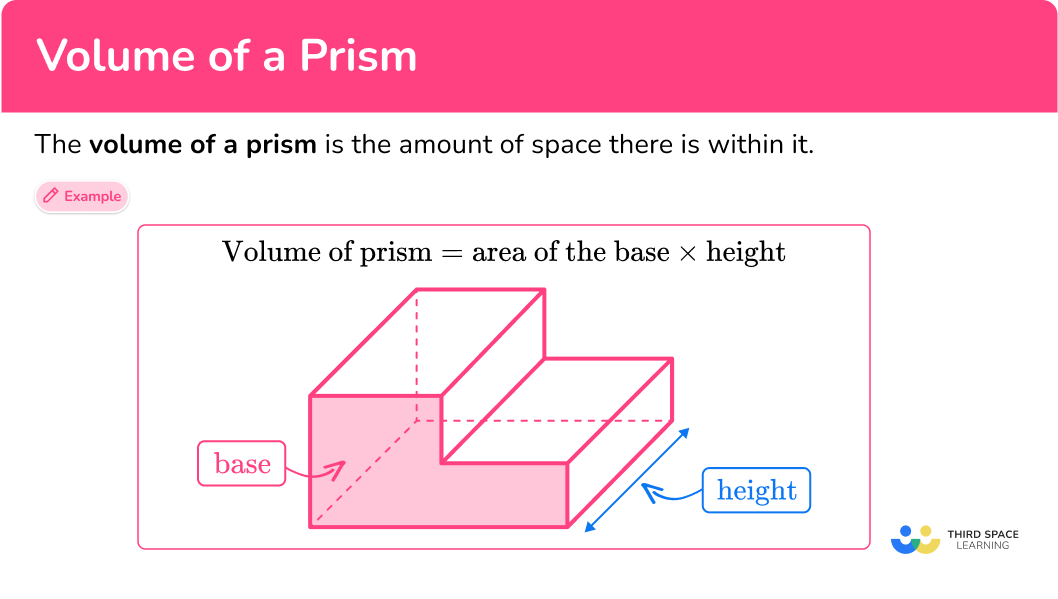## Common Core State Standards

How does this relate to 5 th grade math and 6 th grade math?

• Grade 5 – Geometry (5.G.C.5b)
Apply the formulas V=l \times w \times h and V=b \times h for rectangular prisms to find volumes of right rectangular prisms with whole – number edge lengths in the context of solving real world and mathematical problems.

• Grade 6 – Geometry (6.G.A.2)
Find the volume of a right rectangular prism with fractional edge lengths by packing it with unit cubes of the appropriate unit fraction edge lengths, and show that the volume is the same as would be found by multiplying the edge lengths of the prism.

Apply the formulas V =l \times w \times h and V = b \times h to find volumes of right rectangular prisms with fractional edge lengths in the context of solving real-world and mathematical problems.

• Grade 7 – Geometry (7.G.B.6)
Solve real-world and mathematical problems involving area, volume and surface area of two- and three-dimensional objects composed of triangles, quadrilaterals, polygons, cubes, and right prisms.

## How to calculate the volume of a prism

In order to calculate the volume of a prism:

1. Write down the formula.
2. Calculate the area of the base.
3. Calculate the volume of the prism.
4. Write the answer, including the units.

## Volume of a prism examples

### Example 1: volume of a rectangular prism

A swimming pool is being built in the shape of a rectangular prism.

Calculate the volume of water in the pool when it is completely filled.

1. Write down the formula.

\text {Volume of prism }=\text { Area of the base } \times \text { height}

2Calculate the area of the base.

A rectangular prism has 3 pairs of opposite, congruent faces. Any two opposite, congruent faces can serve as the base, making the height the edge perpendicular to the chosen base. In this case, use the front face as the base.

\begin{aligned} \text{Area of rectangle }&= b\times{h} \\\\ &=12 \times {3} \\\\ &=36\end{aligned}

The area of the rectangle is 36 \mathrm{~m}^2.

3Calculate the volume of the prism.

The height of the prism is 5 \, m.

\begin{aligned} \text{Volume of prism }&= \text{Area of the base }\times{ height}\\\\ &=36\times{5}\\\\ &=180 \end{aligned}

4Write the answer, including the units.

The measurements on this prism are in meters, so the volume is measured in cubic meters.

\text {Volume }=180 \mathrm{~m}^3

### Example 2: volume of a triangular prism

Calculate the volume of the triangular prism:

\text {Volume of prism }=\text { Area of the base } \times \text { height}

A triangular prism has two congruent triangular bases connected by lateral faces – so the base is the triangle.

\begin{aligned} \text{Area of triangle }&=\cfrac{1}{2}\times{b}\times{h}\\\\ &=\cfrac{1}{2}\times{8}\times{3}\\\\ &=12 \end{aligned}

The area of the triangle is 12 \mathrm{~cm}^2.

The height of the prism is 10 \, cm.

\begin{aligned} \text{Volume of prism }&= \text{Area of the base }\times{ height}\\\\ &=12\times{10}\\\\ &=120\\ \end{aligned}

The measurements on this triangular prism are in centimeters, so the volume is measured in cubic centimeters.

\text {Volume }=120 \mathrm{~cm}^3

### Example 3: volume of a cube – fractions

Calculate the volume of the cube:

\text {Volume of prism }=\text { Area of the base } \times \text { height}

\begin{aligned} \text {Area of the square } &=s \times s \\\\ & =7 \, \cfrac{1}{3} \, \times 7 \, \cfrac{1}{3} \\\\ & =\cfrac{22}{3} \, \times \, \cfrac{22}{3} \\\\ & =\cfrac{484}{9} \\\\ & =53 \, \cfrac{7}{9} \end{aligned}

The height of the cube is 7 \, \cfrac{1}{3}.

\begin{aligned} \text {Volume of prism} &= \text {Area of the base} \times height \\\\ & =53 \, \cfrac{7}{9} \, \times 7 \, \cfrac{1}{3} \\\\ & =\cfrac{484}{9} \, \times \cfrac{22}{3} \\\\ & =\cfrac{10,648}{27} \\\\ & =394 \, \cfrac{10}{27}\end{aligned}

No unit is given, so the volume is written with general cubic units.

\text { Volume }=394 \, \cfrac{10}{27} \text { units }^3

### Example 4: volume of a hexagonal prism

Calculate the volume of the prism:

\text { Volume of prism }=\text { Area of the base } \times \text { height }

A hexagonal prism has two congruent hexagonal bases connected by lateral faces – so the base is a hexagon. The area of the hexagon is 50 \, \mathrm{ft}^2.

The height of the prism is 15 \, ft.

\begin{aligned}\text{Volume of prism }&= \text{Area of the base }\times{ height}\\\\ &=50\times{15}\\\\ &=750\end{aligned}

The measurements on this prism are in feet, so the volume is measured in cubic feet.

Volume = 750 \, \mathrm{ft}^3

### Example 5: volume of a compound prism

Calculate the volume of the L-shaped prism:

\text {Volume of prism }=\text { Area of the base } \times \text { height}

To calculate the area of the base, split it into two rectangles and find the missing side lengths. Then calculate the area of each rectangle:

Rectangle A :

\begin{aligned} \text{Area } &=7\times{4}\\\\ &=28 \end{aligned}

Rectangle B :

\begin{aligned}\text{Area } &=6\times{5}\\\\ &=30\end{aligned}

Total area:

28+30=58 \text { inches}^2

The height of the prism is 12 inches.

\begin{aligned} \text{Volume of prism }&= \text{Area of the base }\times{ height}\\\\ &=58\times{12}\\\\ &=696 \end{aligned}

The measurements on this prism are in inches, so the volume is measured in cubic inches.

\text {Volume }=696 \text { inches}^3

### Example 6: missing length in a pentagonal prism

The volume of this prism is 225 \mathrm{~cm}^2 . Calculate the height, h , of the prism:

\text {Volume of prism }=\text { Area of the base } \times \text { height}

A pentagonal prism has two congruent pentagonal bases connected by lateral faces – so the base is a pentagon. The area of the hexagon is 25 \mathrm{~cm}^2.

The volume and the base area are known, so fill them into the equation.

225=25 \times h

The height of the prism, h , times 25 is equal to 225 . Solve the equation to find the value for h that makes the equation true.

\begin{aligned} & 225=25 \times h \\\\ & \div 25 \quad \div 25 \\\\ & 9=h \end{aligned}

The height of the prism is 9 \, cm. Remember, since the height is a side length – it is 2D and therefore measured in units (not units squared or cubed).

### Teaching tips for volume of a prism

• Before having students calculate the volume of prisms on worksheets, make sure they have opportunities to identify and work with physical prisms. This can be through the use of math manipulatives or activities that involve real world prisms.

• Give students a hands-on understanding of volume by having them build different rectangular or composite prisms out of cubes (such as centimeter cubes that come in base 10 block sets or with linking cubes).

### Easy mistakes to make

• Writing the incorrect units or no units at all
Volume is always measured in cubed units. Writing volume as square units or not including any units does not accurately represent what volume is measuring.
For example,
The volume of the prism is…

\bf{36} \bf{36} units \bf{^2} \bf{36} units \bf{^3}
There is no indication of
what is being measured
here… 36 feet? 36 ropes?
36 dogs?

This does not show
volume or any
other unit.

Square units are a
2 -dimensional
measurement for area,
like this rectangle:

This does not show volume.
There are 36 cubed
units that make up
this 3 -dimensional
prism.

This shows volume.

• Calculating with different units
All measurements need to be in the same units before calculating volume.

• Using the incorrect formula
Be careful to apply the correct formula to find the area of the base of the prism – as they are not the same for different shapes. Also make sure that the dimensions used for the height of the prism is perpendicular to the base.

### Practice volume of a prism questions

1. Calculate the volume of the prism: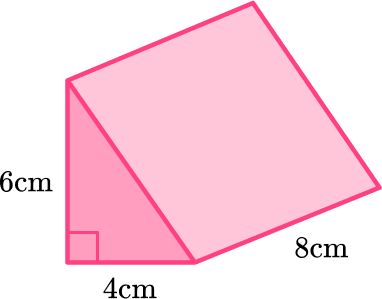192 \mathrm{~cm}^348 \mathrm{~cm}^396 \mathrm{~cm}^340 \mathrm{~cm}^3This is a triangular prism and the bases are two identical triangles.

First calculate the area of the base.

\begin{aligned} \text{Area of triangle }&=\cfrac{1}{2} \, \times 4 \times 6\\\\ &=12 \, \mathrm{cm}^{2} \end{aligned}

Then multiply the area of the base times the height of the prism.

\begin{aligned} \text{Volume of triangular prism }&=12 \times 8\\\\ &=96 \, \mathrm{cm}^{3} \end{aligned}

2. Calculate the volume of the prism: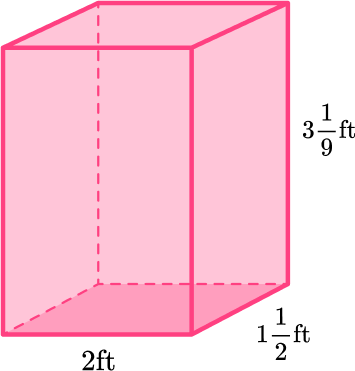6 \cfrac{1}{18} \, \mathrm{ft}^36 \cfrac{5}{9} \, \mathrm{ft}^315 \cfrac{1}{9} \, \mathrm{ft}^315 \cfrac{5}{9} \, \mathrm{ft}^3Any two opposite congruent faces can be used as the base of the rectangular prism. Let’s solve it with the bottom face as the base.

First, calculate the area of the base.

\begin{aligned} \text { Area of rectangle }&= 2 \times 2 \cfrac{1}{2} \\\\ & =\cfrac{2}{1} \, \times \cfrac{5}{2} \\\\ & =\cfrac{10}{2} \\\\ & =5 \, f t^2 \end{aligned}

Then multiply the area of the base times the height of the prism.

\begin{aligned} \text {Volume of rectangular prism } & =5 \times 3 \, \cfrac{1}{9} \\\\ & =\cfrac{5}{1} \, \times \cfrac{28}{9} \\\\ & =\cfrac{140}{9} \\\\ & =15 \, \cfrac{5}{9} \, f t^3 \end{aligned}

3. Calculate the volume of the prism: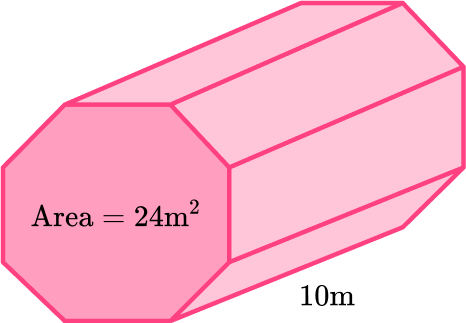240 \mathrm{~m}^32.4 \mathrm{~m}^32,400 \mathrm{~m}^35,760 \mathrm{~m}^3This is a pentagonal prism and the bases are two identical pentagons.

The area of the pentagonal base is given: 24 \mathrm{~m}^2.

The height of the prism is 10 \, m.

\begin{aligned} \text{Volume of prism }&=24 \times 10 \\\\ &=240 \, \mathrm{m}^{3} \end{aligned}

4. Calculate the volume of the prism: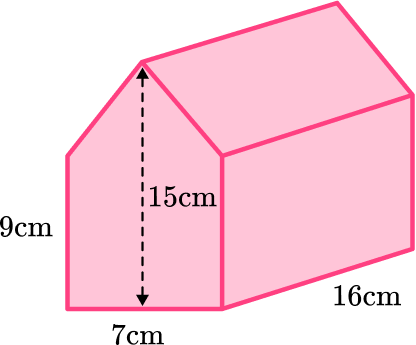84 \mathrm{~cm}^31,008 \mathrm{~cm}^31,008 \mathrm{~cm}^31,344 \mathrm{~cm}^3The base of this pentagonal prism can be broken up into a triangle and a rectangle.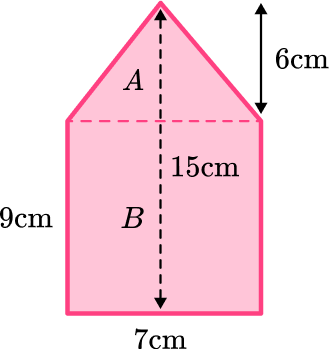Area of triangle A :

\begin{aligned} \text{Area }&=\cfrac{1}{2} \, \times 7 \times 6\\\\ &=21 \, \mathrm{cm}^{2} \end{aligned}

Area of rectangle B :

\begin{aligned} \text{Area }&=7 \times 9 \\\\ &=63 \, \mathrm{cm}^{2} \end{aligned}

\text{Total area: } 21+63=84 \, \mathrm{cm}^{2}

The height of the prism is 16 \, cm.

\begin{aligned} \text{Volume of prism }&=84 \times 16 \\\\ &=1,344 \, \mathrm{cm}^{3} \end{aligned}

5. The volume of this prism is 156 \mathrm{~cm}^3. Find the height, x, of the prism.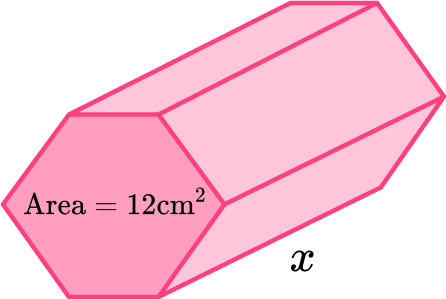1,872 \, cm13 \, cm1.08 \, cm6.5 \, cm\text {Volume of prism }=\text { Area of the base } \times \text { height }

The total volume is 156 \mathrm{~cm}^3 and the area of the base is 12 \mathrm{~cm}^2 – fill those into the formula.

156=12 \, x

The height of the base, x, times 12 is equal to 156. Solve the equation to find the value for x that makes the equation true.

\begin{aligned} & 156=12 x \\\\ & \div 12 \; \div 12 \\\\ & 13=x \end{aligned}

The height of the prism is 13 \, cm. Remember, since the height is a side length – it is 2D and therefore measured in units (not units squared or cubed).

6. The volume of this prism is 270 \, mm^3. Work out the height, h, of the prism.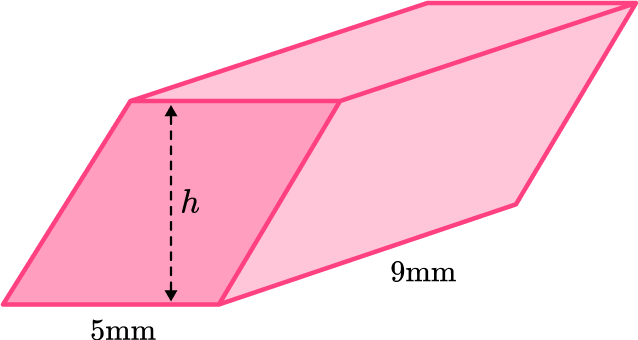12,150 \, mm30 \, mm6 \, mm45 \, mmAll the faces of this prism are parallelograms. Any two opposite congruent faces can be used as the base. Let’s use the bottom face.

\begin{aligned} \text { Area of parallelogram } & =5 \times 9 \\\\ & =45 \end{aligned}

\text {Volume of prism }=\text { Area of the base } \times \text { height }

The total volume is 270 \, mm^3 and area of the base is 45 \, mm^2 – fill those into the formula.

270=45 \times h

The height of the prism, h, times 45 is equal to 270. Solve the equation to find the value for h that makes the equation true.

\begin{aligned} & 270=45 h \\\\ & \div 45 \; \div 45 \\\\ & h=6 \end{aligned}

The height of the prism is 6 \, mm. Remember, since the height is a side length – it is 2D and therefore measured in units (not units squared or cubed).

## Volume of a prism FAQs

Yes, there are many types of prisms that are not included on this page. Any shape that has two identical polygonal bases connected by lateral faces is a prism. The shape of the base of a prism is how it is named. For example, a prism with two congruent trapezoids connected by lateral faces is called a trapezoidal prism.

What is a cuboid?

A cuboid is a 3D shape that has 6 rectangular sides. It is also known as rectangular prism.

How do you calculate the volume of a cylinder?

Even though a cylinder is not a prism, because it has two congruent bases and a consistent height throughout, it is also found by multiplying the area of the base and the height of the cylinder.

## Still stuck?

At Third Space Learning, we specialize in helping teachers and school leaders to provide personalized math support for more of their students through high-quality, online one-on-one math tutoring delivered by subject experts.

Each week, our tutors support thousands of students who are at risk of not meeting their grade-level expectations, and help accelerate their progress and boost their confidence.